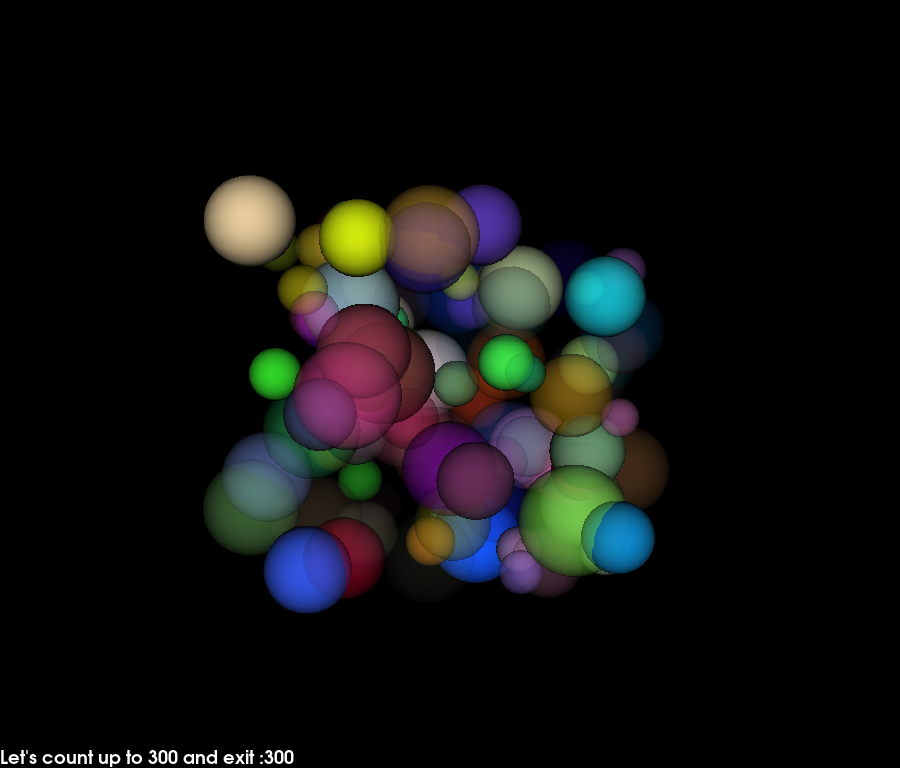# Using a timer¶

This example shows how to create a simple animation using a timer callback.

We will use a sphere actor that generates many spheres of different colors, radii and opacity. Then we will animate this actor by rotating and changing global opacity levels from inside a user defined callback.

The timer will call this user defined callback every 200 milliseconds. The application will exit after the callback has been called 100 times.```import numpy as np
from fury import window, actor, ui
import itertools

xyz = 10 * np.random.rand(100, 3)
colors = np.random.rand(100, 4)

scene = window.Scene()

sphere_actor = actor.sphere(centers=xyz,
colors=colors,

showm = window.ShowManager(scene,
size=(900, 768), reset_camera=False,
order_transparent=True)

showm.initialize()

tb = ui.TextBlock2D(bold=True)

# use itertools to avoid global variables
counter = itertools.count()

def timer_callback(_obj, _event):
cnt = next(counter)
tb.message = "Let's count up to 100 and exit :" + str(cnt)
showm.scene.azimuth(0.05 * cnt)
sphere_actor.GetProperty().SetOpacity(cnt/100.)
showm.render()
if cnt == 100:
showm.exit()